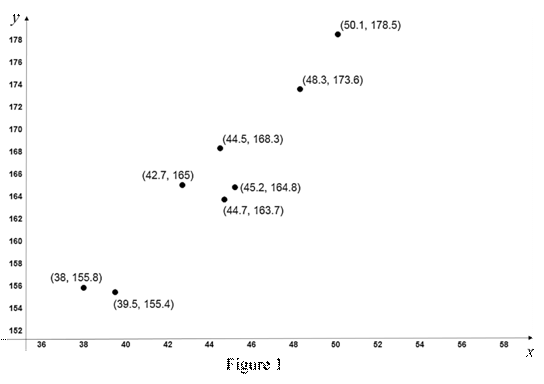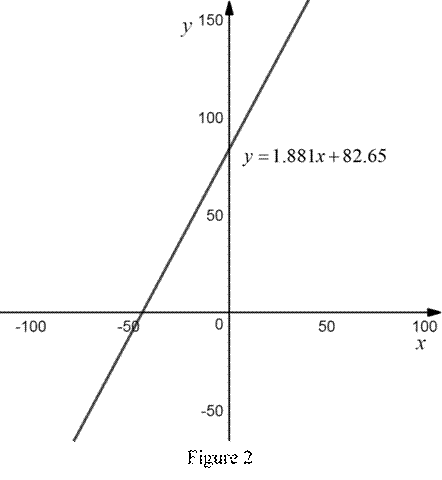# To graph: The scatter plot of the data.### Precalculus: Mathematics for Calcu...

6th Edition
Stewart + 5 others
Publisher: Cengage Learning
ISBN: 9780840068071### Precalculus: Mathematics for Calcu...

6th Edition
Stewart + 5 others
Publisher: Cengage Learning
ISBN: 9780840068071

#### Solutions

Chapter 1, Problem 1P

(a)

To determine

## To graph: The scatter plot of the data.

Expert Solution

### Explanation of Solution

Graph:

Consider the femur length as the x coordinates and height as the y coordinates.

The scatter plots of the given data are shown below in Figure 1.In Figure 1, all the points are plotted on the graph. No two points coincide each other.

(b)

To determine

### To find: The linear function that represents the given data and draw the graph for it.

Expert Solution

The linear function that represents the given data is y=1.881x+82.65 .

### Explanation of Solution

By the use of online calculator, the regression line for the given data obtained is y=1.881x+82.65 , where y is the height and x is the femur length.

The above regression line is in the form of linear equation.

Therefore, the linear function that represents the given data is y=1.881x+82.65 .

The graph that represents the equation y=1.881x+82.65 is shown below in Figure 2.From Figure 2, the graph for the linear equation y=1.881x+82.65 is a straight line.

(c)

To determine

### To find: The height of the person if the femur length is 58 cm.

Expert Solution

The height of the person if the femur length is 58 cm is 191.748 cm.

### Explanation of Solution

Consider the length of the femur is 58 cm.

From part (b), it is obtained that the linear equation that represents the given data is y=1.881x+82.65 .

Substitute x = 58 in the above equation to compute the height.

y=1.881(58)+82.65=109.098+82.65=191.748

Thus, the height of the person if the femur length is 58 cm is 191.748 cm.

### Have a homework question?

Subscribe to bartleby learn! Ask subject matter experts 30 homework questions each month. Plus, you’ll have access to millions of step-by-step textbook answers!